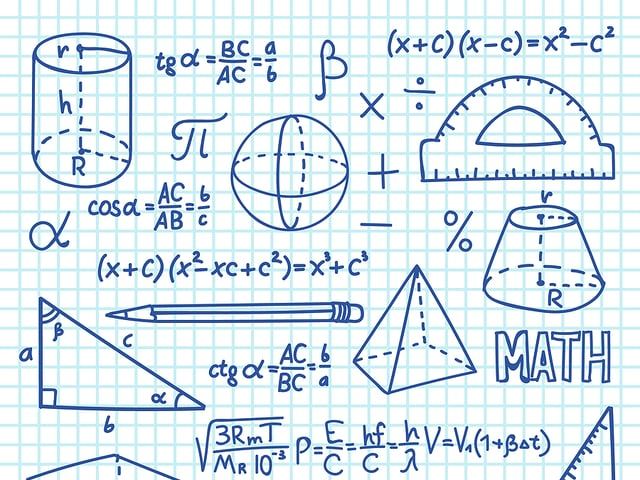# Formulas You’ll Need for the GMAT™ Quantitative Section’s Arithmetic and Geometry Questions

The GMAT™ is a very special kind of test. You will not be able to skip any questions, and the penalty for skipping them is greater than that for getting them wrong. The questions go above and beyond just plugging in values in an equation. You need to be able to think critically, but it would be a very sad scenario if your thought process is hindered by not remembering the proper formula for the problem you’re solving.

Thankfully, we are here to help you! In the following chart, you’ll find the essential arithmetics and geometry formulas you’ll need for the GMAT™ Quantitative Section, so you will not forget them when the test comes. You’ll find formulas relating to the other types of questions on this section of the test here:

Algebra

Statistics and Problem Solving

You can practice for the test by solving our FREE sample test.

## Arithmetic and Geometry Formulas

Category Formula Symbols Comment
Arithmetic $$a+b=b+a$$

$$a \cdot b = b \cdot a$$
a, b = any constant or variable Commutative
Property
Arithmetic $$a+(b+c)=(a+b)+c$$

$$a \cdot (b \cdot c)=(a \cdot b) \cdot c$$
a, b, c = any constant or variable Associative
Property
Arithmetic $$a \cdot (b+c)=a \cdot b + a \cdot c$$ a, b, c = any constant or variable Distributive
Property
Arithmetic $$a+0=a$$ a = any constant or variable Identity Property
Arithmetic $$a \cdot 1 = a$$ a = any constant or variable Identity Property
of Multiplication
Arithmetic $$\dfrac{a}{b} + \dfrac{c}{d} = \dfrac{(a \cdot d)+(c \cdot b)}{(b \cdot d)}$$ a, b, c, d = any real number Remember to simplify
the fraction if
possible.
Arithmetic $$\dfrac{a}{b} \cdot \dfrac{c}{d}=\dfrac{a \cdot c)}{(b \cdot d)}$$ a, b, c, d = any real number Remember to simplify
the fraction if
possible.
Arithmetic $$\dfrac{a}{b} \div \dfrac{c}{d}=\dfrac{a \cdot d)}{(b \cdot c)}$$ a, b, c, d = any real number Remember to simplify
the fraction if
possible.
Arithmetic $$a\dfrac{b}{c}=\dfrac{(a \cdot c)+b}{c}$$ a, b, c = any real number Remember to simplify
the fraction if
possible.
Percents $$a \cdot b\%=a \cdot \dfrac{b}{100}$$ a = any real number
b% = any percent
Remember to simplify
the fraction if
possible.
Percents $$\% = \dfrac{\vert b-a \vert}{b} \cdot 100= \dfrac{c}{b} \cdot 100$$ % = % increase or decrease
a = new value
b = original value
c = amount of change

Geometry $$A=\frac{1}{2} \cdot b \cdot h$$ A = area of triangle
b = base
h = height

Two
Dimensional
Shapes
$$a^2+b^2=c^2$$ a, b = legs of a right triangle
c = hypotenuse of a right triangle

Two
Dimensional
Shapes
$$P=4 \cdot s$$ P = Perimeter of a square
s = side length

Two
Dimensional
Shapes
$$P=(2 \cdot l)+(2 \cdot w)$$ P = Perimeter of a rectangle
l = length
w = width

Two
Dimensional
Shapes
$$A=s^2$$ A = Area of a square
s = side length

Two
Dimensional
Shapes
$$A=l \cdot w$$ A = Area of a rectangle
l = length
w = width

Two
Dimensional
Shapes
$$A=h \cdot \frac{(b_1+b_2)}{2}$$ A = Area of a trapezoid
$$b_n$$ = base n
h = height

Two
Dimensional
Shapes
$$C=2 \cdot \pi \cdot r$$ or $$C=\pi \cdot d$$ C = Circumference of a circle
d = diameter

Two
Dimensional
Shapes
$$S=r \cdot \theta$$ s = arc length
$$\theta$$ = central angle (radians)

Two
Dimensional
Shapes
$$A=\pi \cdot r^2$$ A = Area of a circle

Three
Dimensional
Shapes
$$V=l \cdot w \cdot h$$ V = Volume of a rectangular prism
l = length
w = width
h = height

Three
Dimensional
Shapes
$$SA= \Sigma A_{fi}$$ SA = Surface Area of a prism
$$A_{fi}$$ = Area of face i

Three
Dimensional
Shapes
$$V=\frac{1}{3} \cdot (l \cdot w \cdot h)$$ V = Volume of a pyramid with rectangular base
l = length
w = width
h = height

Three
Dimensional
Shapes
$$SA=\Sigma A_{fi}$$ SA = Surface Area of a pyramid
$$A_{fi}$$ = Area of face i

Three
Dimensional
Shapes
$$V= \pi \cdot r^2 \cdot h$$ V = Volume of a cylinder
h = height

Three
Dimensional
Shapes
$$SA=2B+(C \cdot h)$$ SA = Surface Area of a cylinder
B = Area of the Base
C = Circumference of the Base
h = height

Three
Dimensional
Shapes
$$V= \frac{4}{3} \cdot \pi \cdot r^3$$ V = Volume of a sphere
$$SA=4 \cdot \pi \cdot r^2$$ SA = Surface Area of a sphere